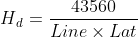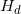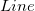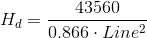# Sprinkler Density

This calculator estimates the number of sprinklers required per acre based on different spacing. Lateral spacing is the distance between sprinkler heads in the lateral, while line spacing measures the distance between the lateral sets, or the distance between sprinkler heads in the other direction from the lateral spacing measurements. For triangular spacing, the distance between sprinkler heads is the same distance between all nearest sprinklers (equilateral triangles). The head density is the number of sprinkler heads per area. Learn more about the units used on this page.

Lateral Spacing:
Line Spacing:

Line Spacing:

## The Equations

These calculators use these formulas to determine sprinkler density

#### Rectangular SpacingWhere:= Sprinkler Head Density (heads/acre)= Line Spacing (ft)= Lateral Spacing (ft)

#### Triangular SpacingWhere:= Sprinkler Head Density (heads/acre)= Lateral Spacing (ft)= Line Spacing (ft)